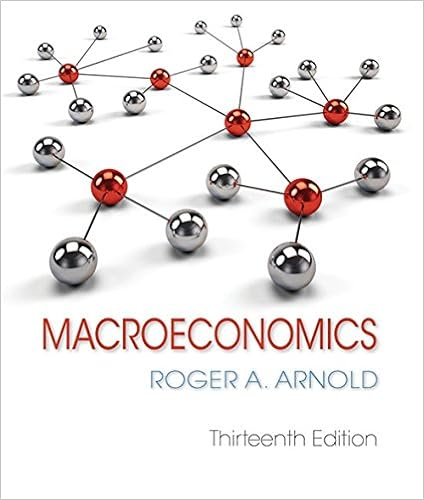# Correct mark 100 out of 100 flag question question

• Test Prep
• 38
• 91% (32) 29 out of 32 people found this document helpful

This preview shows page 3 - 7 out of 38 pages.

##### We have textbook solutions for you!
The document you are viewing contains questions related to this textbook.The document you are viewing contains questions related to this textbook.
Chapter 9 / Exercise 5
Macroeconomics
Roger A. ArnoldExpert Verified
Question 7 Correct Mark 1.00 out of 1.00 Flag question Question text Assume that individuals do not hold currency (i.e., c= 0). If the ratio of reserves to deposits is 0.3, the money multiplier is: Select one: a. 5.33. b. 1.33. c. 0.33.
##### We have textbook solutions for you!
The document you are viewing contains questions related to this textbook.The document you are viewing contains questions related to this textbook.
Chapter 9 / Exercise 5
Macroeconomics
Roger A. ArnoldExpert Verified
d. 3.33. e. 2.33. Feedback The correct answer is: 3.33.
Question 8 Correct Mark 1.00 out of 1.00 Flag question Question text The equilibrium condition in the goods market holds that:
Question 9 Correct Mark 1.00 out of 1.00 Flag question Question text An increase in the propensity to save from 0.36 to 0.56 will cause:
Question 10 Incorrect Mark 0.00 out of 1.00 Flag question Question text If individuals do not hold currency, we know that:
.
Question 11 Correct Mark 1.00 out of 1.00 Flag question Question text Which of the following generally occurs when a central bank pursues contractionary monetary policy? Select one: a. The central bank purchases bonds and the interest rate increases. b. The central bank sells bonds and the interest rate increases. c. The central bank sells bonds and the interest rate decreases. d. The central bank sells reserves and the interest rate increases. e. The central bank purchases bonds and the interest rate decreases. Feedback
The correct answer is: The central bank sells bonds and the interest rate increases.
•••